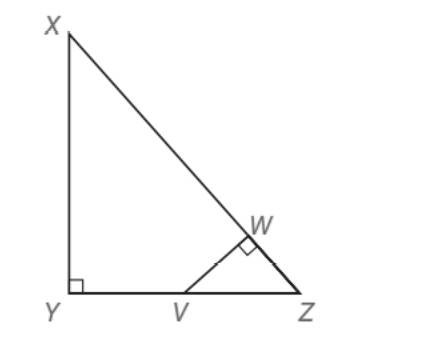Chapter 5.5, Problem 37EElementary Geometry For College St...

7th Edition
Alexander + 2 others
ISBN: 9781337614085

Solutions

Chapter
SectionElementary Geometry For College St...

7th Edition
Alexander + 2 others
ISBN: 9781337614085
Textbook Problem

In right triangle X Y Z , X Y = 3 and Y Z = 4 . If V is the midpoint of Y Z ¯ and m ∠ V W Z = 90 ° , find V W .(HINT: Draw X V ¯ . )To determine

To find:

VW, in the right triangle XYZ, XY=3 and YZ=4. V is the midpoint of YZ and

mVWZ=90°

Explanation

Approach:

Pythagorean Theorem.

In any right triangle, the square of the longer side is equal to the sum of the squares of the other two legs of the triangle.

For the right triangle XYZ with right angle at Z,

XY2=XZ2+YZ2.

Calculation:

Given,

A right triangle XYZ with

XY=3

YZ=4

V is the midpoint of YZ and mVWZ=90°

Since V is the midpoint of YZ

VY¯VZ¯

Thus,

VY=VZ=12·YZ

=12·4

VY=VZ=2 units.

Draw an auxiliary line segment XV¯ joining X and V.

Apply Pythagorean theorem is the right triangle XYV.

XV2=XY2+YV2

=32+22

=9+4

XV2=13

Apply square root on both sides,

XV=13 units.

Also, in the right XYZ

XZ2=XY2+YV2

=32+42

=9+16

XZ2=25

Apply square root on both sides,

XZ=5 units.

Now, in the right VWZ,

VZ2=VW2+WZ2

(2)2=VW2+WZ2 . . . (1)

Again, in the right XWV,

XZ2=XW2+VW2

(13)2=XW2+VW2

13=XW2+VW2 . . . (2)

Since XZ=5 we have

XZ=5

XW+WZ=5

Add; -WZ on both sides,

XW+WZ+-WZ=5+(-WZ)

XW+( WZ+-WZ=5+(-WZ)

XW=5-W

Still sussing out bartleby?

Check out a sample textbook solution.

See a sample solution

The Solution to Your Study Problems

Bartleby provides explanations to thousands of textbook problems written by our experts, many with advanced degrees!

Get Started

In Exercises 75-98, perform the indicated operations and/or simplify each expression. 86. (5x + 2)(3x 4)

Applied Calculus for the Managerial, Life, and Social Sciences: A Brief Approach

Solve the equations in Exercises 112 for x (mentally, if possible). 4x5=8

Finite Mathematics and Applied Calculus (MindTap Course List)

Simplify: 112128

Elementary Technical Mathematics

The graph of is: a) b) c) d)

Study Guide for Stewart's Multivariable Calculus, 8th